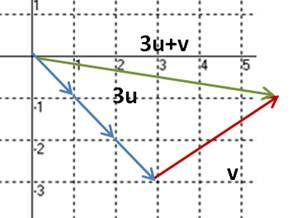### Sample Problem

Given that u=i-j and v=3i+2j, which of the following vectors represents 3u+v?#### Solution

u=i-j=<1,-1>

v=3i+2j=<3, 2>

3u+v=3<1,-1>+<3, 2>=<3, -3>+<3, 2>

=<6, -1>:

the resultant vector has an x-component of 6 and a y-component of -1, vector (C).